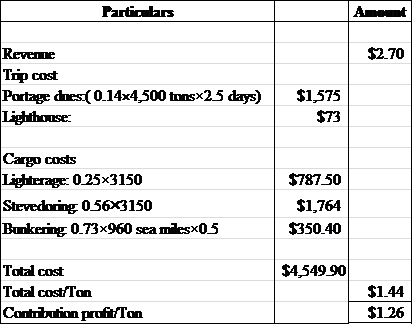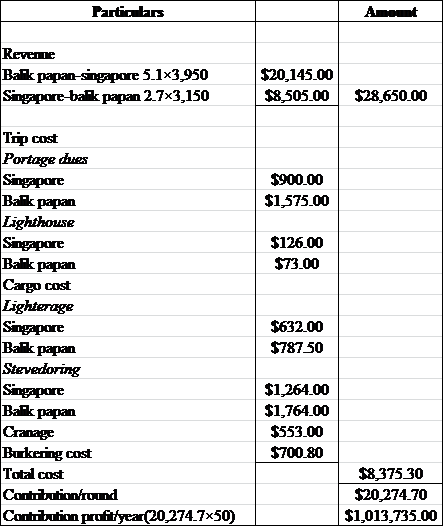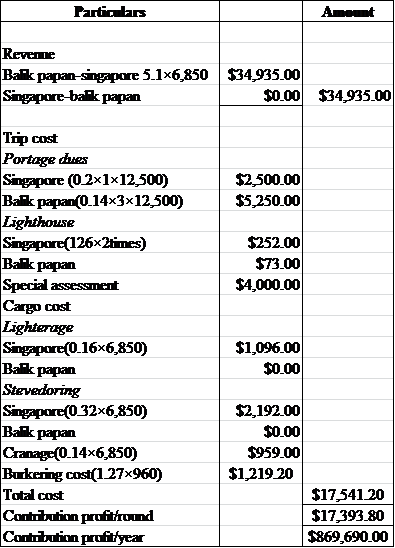# Cases in Cost Management

## Quiz 30 : TashtegoLooking for Management Homework Help?

## Quiz 30 :Tashtego

Question TypeThe issue in this case is very exotic-should the motor Vessel Tashtego be used on the tapioca run between Singapore and Balik Papan in East Asia or as a freight tender between Zanzibar and Dar-es-Salaam in East Africa This decision hinges on a cost analysis as to which option is more profitable. One issue we will discuss in class is what constitutes a "variable" cost when some costs vary per ton, some per day, some per mile, and some per stop. Other issues we will discuss include the importance of defining the alternatives precisely, the concept of profit contribution per unit of capacity, and the role of cost analysis in helping management to ask the right questions. In order to help you work through this difficult but also valuable case, the following specific questions should be answered in order. These questions help you develop, piece by piece, an overall analysis of the decision. Try to imagine tackling the case with no specific questions as a guide! How much profit contribution can be earned by carrying 1 ton of tapioca from Balik Papan to Singapore, dock to dock, considering revenue and cargo costs How much can be earned by carrying 1 ton of general merchandise from Singapore to Balik Papan
Free
Essay

Profit contribution that can be earned can be calculated as follows:
Profit contribution : It is the contribution calculated by finding out the difference of the total costs from the revenue.
Referring exhibits 1 and 2 the contribution can be summarized as below:
The following spreadsheet shows the calculation of the contribution :Thus, the profit contribution that can be earned by carrying 1 ton of tapioca is $1.26. The profit contribution of carrying 1 ton of merchandise cannot be estimated as full information regarding the portage sues and the cargo cost for the same is not mentioned. TagsThe issue in this case is very exotic-should the motor Vessel Tashtego be used on the tapioca run between Singapore and Balik Papan in East Asia or as a freight tender between Zanzibar and Dar-es-Salaam in East Africa This decision hinges on a cost analysis as to which option is more profitable. One issue we will discuss in class is what constitutes a "variable" cost when some costs vary per ton, some per day, some per mile, and some per stop. Other issues we will discuss include the importance of defining the alternatives precisely, the concept of profit contribution per unit of capacity, and the role of cost analysis in helping management to ask the right questions. In order to help you work through this difficult but also valuable case, the following specific questions should be answered in order. These questions help you develop, piece by piece, an overall analysis of the decision. Try to imagine tackling the case with no specific questions as a guide! Given the contribution/ton figures arrived at in question 1, what is the total contribution which can be earned on one round trip by Tashtego between Singapore and Balik Papan and return By one of the large vessels Free Essay Answer: Answer: The contribution that can be earned on one round trip by Tashtego between Singapore and Balik Papan can be calculated as follows: Profit contribution: It is the contribution that is calculated by finding out the difference of the total costs from the revenue. The following spreadsheet shows the calculation of the total contribution:Thus, the profit contribution that can be earned is$20274.7.

TagsThe issue in this case is very exotic-should the motor Vessel Tashtego be used on the tapioca run between Singapore and Balik Papan in East Asia or as a freight tender between Zanzibar and Dar-es-Salaam in East Africa This decision hinges on a cost analysis as to which option is more profitable. One issue we will discuss in class is what constitutes a "variable" cost when some costs vary per ton, some per day, some per mile, and some per stop. Other issues we will discuss include the importance of defining the alternatives precisely, the concept of profit contribution per unit of capacity, and the role of cost analysis in helping management to ask the right questions. In order to help you work through this difficult but also valuable case, the following specific questions should be answered in order. These questions help you develop, piece by piece, an overall analysis of the decision. Try to imagine tackling the case with no specific questions as a guide! Independent of the amount Slid type of cargo carried, what are the incremental trip costs of sending Tashtego on a round trip between Singapore and Balik Papan One of the large vessels
Free
Essay

The incremental trip cost of Tashtego ship on the round trip between Singapore and Balik papan on a large vessel can be calculated using the exhibits 2 and 3.
The following spreadsheet shows the calculation of incremental trip cost :Thus, the cost per round is $17,541.20 and contribution profit per year is$869,690.

TagsThe issue in this case is very exotic-should the motor Vessel Tashtego be used on the tapioca run between Singapore and Balik Papan in East Asia or as a freight tender between Zanzibar and Dar-es-Salaam in East Africa This decision hinges on a cost analysis as to which option is more profitable. One issue we will discuss in class is what constitutes a "variable" cost when some costs vary per ton, some per day, some per mile, and some per stop. Other issues we will discuss include the importance of defining the alternatives precisely, the concept of profit contribution per unit of capacity, and the role of cost analysis in helping management to ask the right questions. In order to help you work through this difficult but also valuable case, the following specific questions should be answered in order. These questions help you develop, piece by piece, an overall analysis of the decision. Try to imagine tackling the case with no specific questions as a guide! Considering revenue, trip costs, and cargo costs what is the total contribution per round trip for each of the vessel types What is the total contribution per year for each of the vessel types
Essay